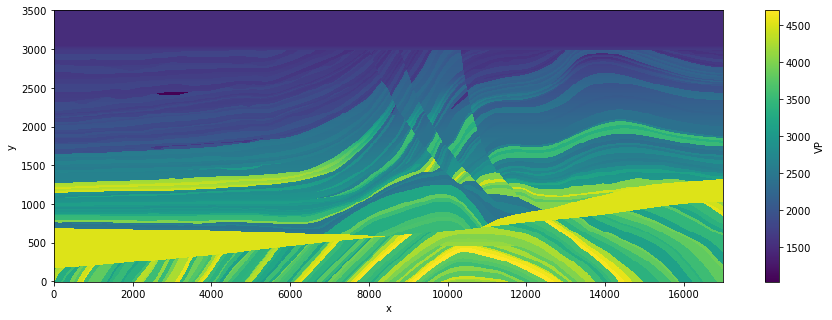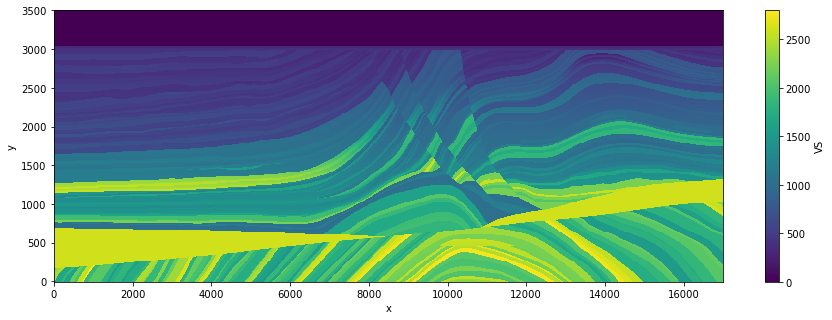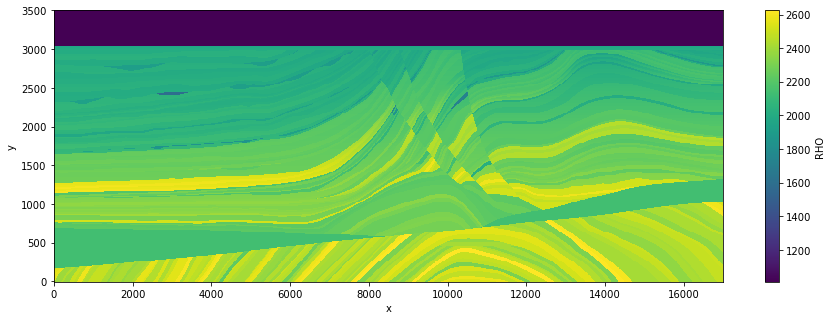Version:

This documentation is not for the latest stable Salvus version.This tutorial is presented as Python code running inside a Jupyter Notebook, the recommended way to use Salvus. To run it yourself you can copy/type each individual cell or directly download the full notebook, including all required files.# Marmousi Model

## Introduction

The AGL Elastic Marmousi model is the most recent generation of the classic Marmousi seismic benchmark. In this tutorial we will cover how one can use this model within Salvus. In particular, we will cover:
• How to read a SEG-Y file using Obspy
• How to read in a rectilinear model using xarray
• How to interpolate a 2-D regularly-gridded model onto the mesh
• How to generate a synthetic seismic shotgather using the model

## Environment setup

As always, our first job is to import the relevant Python packages into our notebook.
Copy
%matplotlib inline
%config Completer.use_jedi = False

import numpy as np
import os
import shutil
import pathlib
import requests
import time
import xarray as xr

import salvus.namespace as sn
from salvus.namespace import simple_config as sc

SALVUS_FLOW_SITE_NAME = os.environ.get("SITE_NAME", "local")
SIMULATION_MAX_FREQUENCY = 5.0

## Model building

Information about the Marmousi model we'll be using can be found here. The model is stored as a SEG-Y file, and the next cell will download and unpack the model for you. Note that the model file is relatively large (~150 MB), so make sure you have enough disk space before continuing.
# Download Marmousi model ~150 MB.
target_path = "elastic-marmousi-model.tar.gz"
if not pathlib.Path(target_path).exists():
url = (
"https://s3.amazonaws.com/open.source.geoscience/open_data"
"/elastic-marmousi/elastic-marmousi-model.tar.gz"
)

response = requests.get(url, stream=True)
if response.status_code == 200:
with open(target_path, "wb") as f:
shutil.unpack_archive(target_path)

With our environment is set up, and the model downloaded, we can begin building the Salvus mesh. Most material models in seismic exploration are discretized onto a regular grid, and the open-source SalvusPythonToolkit provides a simple interface to create two or three dimensional Salvus meshes based on such models. As models can be stored in a wide variety of format such as SEG-Y, or RSF, we use xarray as an intermediary to encapsulate both the model parameters and geometry. More info on xarray, including extensive documentation, can be found here. Reading our Marmousi model into an xarray.Dataset is trivial, and the process can be inspected by opening the acompanying getting_started_tools.py file.
In general, if you are working working with a regularly gridded model in either two or three dimensions, a recipie similar to the function provided should be all you need to do to get your model into a form Salvus Mesh can understand. All that is required is an xarray dataset with the following information
• The coordinate values in meters (named 'x', 'y', and optionally 'z')
• The material parameters in SI units, with names following the parameter naming convention
# Read and plot marmousi model.
model_directory = pathlib.Path("./elastic-marmousi-model/model")
model_directory, ["VP", "RHO", "VS"]
)
A nice feature of xarray.Dataset object is the ability to quickly plot the contained data. Let's do this below as a way to quickly QC that the model reading went correctly.
marmousi["VP"].transpose("y", "x").plot(aspect="auto", figsize=(15, 5))
marmousi["VS"].transpose("y", "x").plot(aspect="auto", figsize=(15, 5))
marmousi["RHO"].transpose("y", "x").plot(aspect="auto", figsize=(15, 5))
<matplotlib.collections.QuadMesh at 0x7fd0f8c68d10>Note that the top layer models a fluid ocean. In order to accurately incorporate the interface of the ocean bottom into the spectral-element mesh. We will first cut out this part from the model and later on add it back as an ocean layer. This will ensure that the interface conditions between solid and fluid media are correctly imposed and that the model is indeed discontinuous across this interface.
marmousi_elastic = marmousi.where(marmousi["y"] <= 3500 - 37 * 12.5, drop=True)
marmousi_elastic["VP"].transpose("y", "x").plot(aspect="auto", figsize=(15, 5))
marmousi_elastic["VS"].transpose("y", "x").plot(aspect="auto", figsize=(15, 5))
marmousi_elastic["RHO"].transpose("y", "x").plot(
aspect="auto", figsize=(15, 5)
)
<matplotlib.collections.QuadMesh at 0x7fd0f967a950>